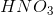# SAT II Chemistry : Oxidation-Reduction Reactions

## Example Questions

### Example Question #1 : Oxidation State

What is the oxidation number of nitrogen in?Explanation:

First, note that the molecule does not have a charge, meaning that the oxidation numbers of each atom must add up to zero. Hydrogen has an oxidation number ofand oxygen has an oxidation number of. Thus, if we call the oxidation number of nitrogen, we can get the equation. Solving this gives, so the oxidation number of nitrogen isin this molecule.

### Example Question #1 : Reaction Types

The following is a modified true/false question. In it, you must decide if each individual statement is true (T) or false (F). If both are true, then you must also decide if the second statement is a correct explanation (CE) of the first statement.

I: Anion would undergo reduction to formmetal

BECAUSE

II: reduction is a loss of electrons

T, F

T, T (Statement II is not a correct explanation of Statement I)

T, T, CE

F, T

F, F